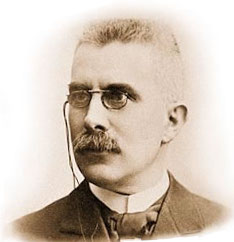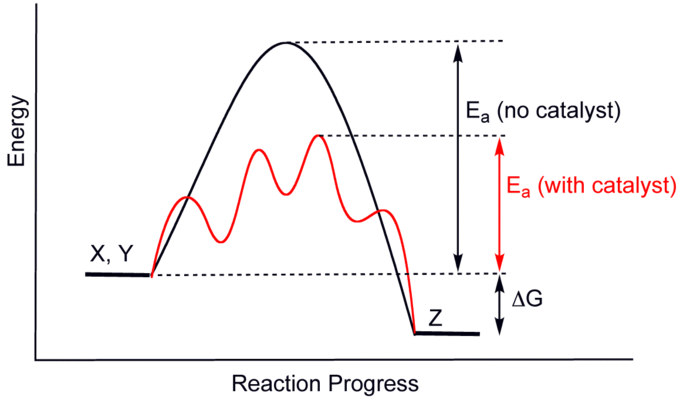Le Chatelier’s Principle

Le Chatelier’s principle states that changes to an equilibrium system will result in a predictable shift that will counteract the change.

Learning Objectives

Recall factors that Le Chatelier’s principle states will affect the equilibrium of a system

Key Takeaways

Key Points

• Le Chatelier’s principle can be used to predict the behavior of a system due to changes in pressure, temperature, or concentration.
• Le Chatelier’s principle implies that the addition of heat to a reaction will favor the endothermic direction of a reaction as this reduces the amount of heat produced in the system.
• Increasing the concentration of reactants will drive the reaction to the right, while increasing the concentration of products will drive the reaction to the left.

Key Terms

• equilibrium: The state of a reaction in which the rates of the forward and reverse reactions are the same.
• collision theory: Relates collisions among particles to reaction rate; reaction rate depends on factors such as concentration, surface area, temperature, stirring, and the presence of either a catalyst or an inhibitor.

Le Chatelier’s principle is an observation about chemical equilibria of reactions. It states that changes in the temperature, pressure, volume, or concentration of a system will result in predictable and opposing changes in the system in order to achieve a new equilibrium state. Le Chatelier’s principle can be used in practice to understand reaction conditions that will favor increased product formation. This idea was discovered and formulated independently by Henri Louis Le Chatelier and Karl Ferdinand Braun.Henry Le Chatelier: A photograph of Henry Le Chatelier.

Changes in Concentration

According to Le Chatelier’s principle, adding additional reactant to a system will shift the equilibrium to the right, towards the side of the products. By the same logic, reducing the concentration of any product will also shift equilibrium to the right.

The converse is also true. If we add additional product to a system, the equilibrium will shift to the left, in order to produce more reactants. Or, if we remove reactants from the system, equilibrium will also be shifted to the left.

Thus, according to Le Chatelier’s principle, reversible reactions are self-correcting; when they are thrown out of balance by a change in concentration, temperature, or pressure, the system will naturally shift in such a way as to “re-balance” itself after the change.

This can be illustrated by the equilibrium of this reaction, where carbon monoxide and hydrogen gas react to form methanol:

$\text{CO} + 2 \text{H}_2 \rightleftharpoons \text{CH}_3\text{OH}$

Suppose we were to increase the concentration of CO in the system. By Le Chatelier’s principle, we can predict that the amount of methanol will increase, thereby decreasing the total change in CO. If we add a species to the overall reaction, the reaction will favor the side opposing the addition of the species. Likewise, the subtraction of a species would cause the reaction to fill the “gap” and favor the side where the species was reduced.

This observation is supported by the collision theory. As the concentration of CO is increased, the frequency of successful collisions of that reactant would increase as well, allowing for an increase in the forward reaction, and thus the generation of the product. Even if a desired product is not thermodynamically favored, the end-product can be obtained if it is continuously removed from the solution.

Changes in Pressure

A change in pressure or volume will result in an attempt to restore equilibrium by creating more or less moles of gas. For example, if the pressure in a system increases, or the volume decreases, the equilibrium will shift to favor the side of the reaction that involves fewer moles of gas. Similarly, if the volume of a system increases, or the pressure decreases, the production of additional moles of gas will be favored.

Consider the reaction of nitrogen gas with hydrogen gas to form ammonia:

$\text{N}_2 + 3 \text{H}_2 \rightleftharpoons 2 \text{NH}_3\quad\quad \Delta \text{H}=-92\;\text{kJ mol}^{-1}$

Note the number of moles of gas on the left-hand side and the number of moles of gas on the right-hand side. When the volume of the system is changed, the partial pressures of the gases change. If we were to decrease pressure by increasing volume, the equilibrium of the above reaction would shift to the left, because the reactant side has greater number of moles than the product side. The system tries to counteract the decrease in partial pressure of gas molecules by shifting to the side that exerts greater pressure.

Similarly, if we were to increase pressure by decreasing volume, the equilibrium would shift to the right, counteracting the pressure increase by shifting to the side with fewer moles of gas that exert less pressure.

Lastly, for a gas-phase reaction in which the number of moles of gas on both sides of the equation are equal, the system will be unaffected by changes in pressure, since $\Delta \text{n} =0$.

What would happen to the equilibrium position of the reaction if an inert gas, such as krypton or argon, were added to the reaction vessel? Answer: nothing at all. Remember that the system will always shift so that the ratio of products and reactants remains equal to Kp or Kc. An inert gas will not react with either the reactants or the products, so it will have no effect on the product/reactant ratio, and therefore, it will have no effect on equilibrium.

Changes in Temperature

The effect of temperature on equilibrium has to do with the heat of reaction. Recall that for an endothermic reaction, heat is absorbed in the reaction, and the value of $\Delta \text{H}$ is positive. Thus, for an endothermic reaction, we can picture heat as being a reactant:

$\text{heat}+\text{A}\rightleftharpoons \text{B}\quad \Delta \text{H}=+$

For an exothermic reaction, the situation is just the opposite. Heat is released in the reaction, so heat is a product, and the value of $\Delta \text{H}$ is negative:

$\text{A}\rightleftharpoons \text{B}+\text{heat}\quad\Delta \text{H}=-$

If we picture heat as a reactant or a product, we can apply Le Chatelier’s principle just like we did in our discussion on raising or lowering concentrations. For instance, if we raise the temperature on an endothermic reaction, it is essentially like adding more reactant to the system, and therefore, by Le Chatelier’s principle, the equilibrium will shift the right. Conversely, lowering the temperature on an endothermic reaction will shift the equilibrium to the left, since lowering the temperature in this case is equivalent to removing a reactant.

For an exothermic reaction, heat is a product. Therefore, increasing the temperature will shift the equilibrium to the left, while decreasing the temperature will shift the equilibrium to the right.

Example

In which direction will the equilibrium shift if the temperature is raised on the following reaction?

$\text{N}_2\text{O}_4(\text{g}) \rightleftharpoons 2\text{NO}_2(\text{g})\quad \Delta \text{H}=+57.2$

Our heat of reaction is positive, so this reaction is endothermic. Since this reaction is endothermic, heat is a reactant. By Le Chatelier’s principle, increasing the temperature will shift the equilibrium to the right, producing more NO2.

Le Chatelier’s principle: This lesson shows how Le Chatelier’s principle predicts changes in an equilibrium. It also demonstrates an easy and convenient method for making predictions about the effects of temperature, concentration, and pressure.

The Effect of a Catalyst

Catalysts speed up the rate of a reaction, but do not have an affect on the equilibrium position.

Learning Objectives

Evaluate the effect of a catalyst on a chemical equilibrium

Key Takeaways

Key Points

• Catalysts are compounds that accelerate the rate of a reaction.
• Catalysts accelerate reactions by reducing the energy of the rate-limiting transition state.
• Catalysts do not affect the equilibrium state of a reaction.

Key Terms

• transition state: An intermediate state in a chemical reaction that has a higher free energy than both the reactants the products.

The Effect of a Catalyst on Equilibrium

Reactions can be sped up by the addition of a catalyst, including reversible reactions involving a final equilibrium state. Recall that for a reversible reaction, the equilibrium state is one in which the forward and reverse reaction rates are equal. In the presence of a catalyst, both the forward and reverse reaction rates will speed up equally, thereby allowing the system to reach equilibrium faster. However, it is very important to keep in mind that the addition of a catalyst has no effect whatsoever on the final equilibrium position of the reaction. It simply gets it there faster.

Recall that catalysts are compounds that accelerate the progress of a reaction without being consumed. Common examples of catalysts include acid catalysts and enzymes. Catalysts allow reactions to proceed faster through a lower-energy transition state. By lowering the energy of the transition state, which is the rate-limiting step, catalysts reduce the required energy of activation to allow a reaction to proceed and, in the case of a reversible reaction, reach equilibrium more rapidly.Catalysis: A catalyst speeds up a reaction by lowering the activation energy required for the reaction to proceed.

To reiterate, catalysts do not affect the equilibrium state of a reaction. In the presence of a catalyst, the same amounts of reactants and products will be present at equilibrium as there would be in the uncatalyzed reaction. To state this in chemical terms, catalysts affect the kinetics, but not the thermodynamics, of a reaction. If the addition of catalysts could possibly alter the equilibrium state of the reaction, this would violate the second rule of thermodynamics; we would be getting “something for nothing,” which is physically impossible.

Interactive: Catalysis: The model contains reactants which will form the reaction: A₂ + B₂ –> 2 AB. In this case the model has been set so the activation energy is high. Try running the reaction with and without a catalyst to see the effect catalysts have on chemical reactions. 1. Run the model to observe what happens without a catalyst. 2. Pause the model. 3. Add a few (3 – 4) catalyst atoms to the container by clicking the button. 4. Run the model again, and observe how the catalyst affects the reaction.